# Team:NJU NJUT China/modeling

main## MODEL

Background:

Genome-editing nucleases are valuable for understanding genes’ functions and potential gene therapy applications. Cas9, normally programmed by a dual RNA consisting of the crRNA and tracrRNA, , is in a sense capable of valuable editing nuclease. The core components of it can be combined into a single hybrid ‘guide RNA’ for Cas9 targeting. Using the CRISPR/Cas strategy, retargeting the nuclease complex requires nothing but introduction of a new RNA sequence. There is no need to reengineer the specificity of protein transcription factors, which is the major advantage of the system and the strategy. Two new studies have recently adapted this system to achieve genome editing in mammalian cells.

However, in order to understand the essence of the mechanism as well as to take advantage of the CRISPR/Cas system for any kind of application including gene-editing or antiviral improvement.et al, there are still some questions necessary to be discussed. Why CRISPR/Cas systems use these sequences to be the guider for site-specific cleavage? What kinds of properties these sequences have in common? And what can we learn from these naturally-selected spacers to be the references when we artificially design the new RNA sequence for targeting?

Objective:

To find out what properties these sequences share with each other, we analyzed hundreds of spacers and developed a mathematical model that describes the influences between the bases in the single strand RNA (gRNA). We applied this model to search the most likely sequences to be the pro-spacer, and to improve the efficiency and precision of the Cas9-cleavage.

Model Creator: Jialiang Ni (also the coder), Ruitao Jin, Xi min.

Theoretical Strategy

The core of the theoretical strategy is to describe the interactions between nucleotides. More specifically, how can we analyze the influence of given oligonucleotide (including mononucleotide, dinucleotide, trinucleotide or tetranucleotide) on the following nucleotide quantificationally? We use the probability of the occurrence of A, T, C, G, respectively, after the mononucleotide (totally 4, A, T, G, C), dinucleotide (totally 16, such as AA, AT, AC, AG, etc.), trinucleotide (totally 64, such as AAA, AAT, AAC, AAG, ATA, etc.) and tetranucleotide (totally 256), to show the interactions among nucleotides.

Before the function is given, these probability shall be calculated and shown as follow:

Obviously, we can notice that tetranucleotide have the most significant influence on the following nucleotide. We next build a function to measure these interactions quantificationally.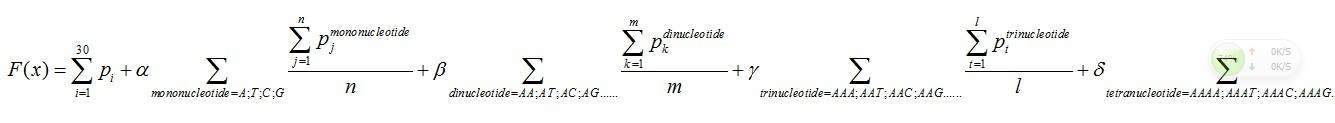There are five parts in this function.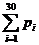Pi means the probability, which is calculated from our database shown above, of the existing nucleotide (A, T, C,
G) in the sequence tested occurring at specific sites. For example, if the first nucleotide in the sequence tested is A, and the probability calculated of the occurrence of A at that site is 25%, Pi=0.25.Pj means the probability, which is calculated from our database shown above, of the existing nucleotide (A, T, C, G) occurring after specific mononucleotide. For example, if we found A show once after the mononucleotide A in the sequence tested and the probability calculated of the occurrence of A after A is 25%, Pj=0.25. Then we should add a 0.25 into this function. n means the number we found in the sequence tested, of specific mononucleotide (A, T, C, G).Pk means the probability, which is calculated from our database shown above, of the existing nucleotide (A, T, C, G) occurring after specific dinucleotide. For example, if we found A show once after the dinucleotide AA in the sequence tested and the probability calculated of the occurrence of A after AA is 25%, Pk=0.25. Then we should add 0.25 into this function. m means the number we found in the sequence tested, of the specific dinucleotide (AA, AT, AC, etc.).Pt means the probability, which is calculated from our database shown above, of the existing nucleotide (A, T, C, G) occurring after specific trinucleotide. For example, if we found A show once after the trinucleotide AAA in the sequence tested and the probability calculated of the occurrence of A after AAA is 25%, Pt=0.25. Then we should add 0.25 into this function. l means the number we found in the sequence tested, of the specific trinucleotide (AAA, AAT, AAC, etc.).Ps means the probability, which is calculated from our database shown above, of the existing nucleotide (A, T, C, G) occurring after specific tetranucleotide. For example, if we found A show once after the tetranucleotide AAAA in the sequence tested and the probability calculated of the occurrence of A after AAAA is 25%, Ps=0.25. Then we should add 0.25 into this function. q means the number we found in the sequence tested, of the specific tetranucleotide (AAAA, AAAT, AAAC, etc.).

Each sequence has its own characteristic coordinates based on those calculation, for example, if we get the result of these five parts as follow: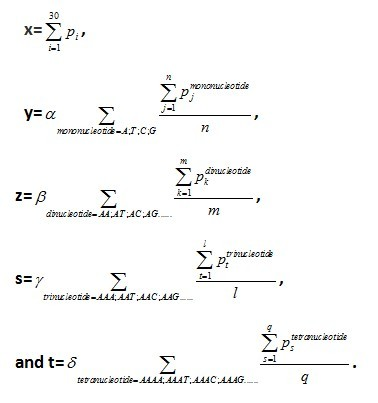Then we get the coordinates (x, y, z, s. t) of this sequence.

We test all 30nt spacer of Streptococcus thermophiles from the CRISPR Database(http://crispr.u-psud.fr/Server/CRISPRfinder.php?page=Help), and get 221 coordinates in total.

To guarantee the variance of every function value when plugging in the coordinates into the function is small enough. We use the Monte Carlo Method to assign the coefficient of each part. During the process, the algorithm run 100,000 cycles. Then we get the function as follow: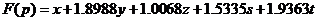To our surprise, we get result below after a statistical analysis: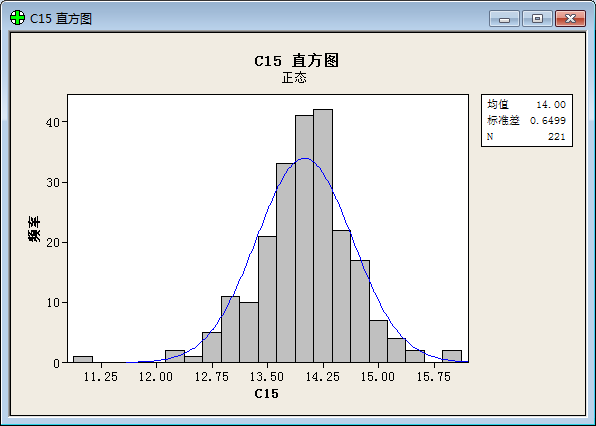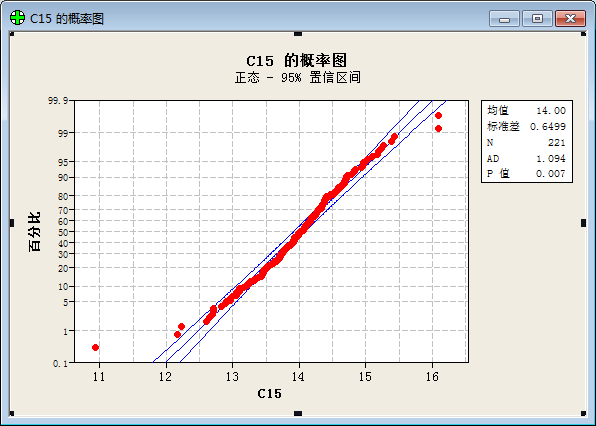(“频率”=frequency; “直方图”= histogram; “正态”= Normal Distribution; “均值”=Average value; “标准差”=Standard variance; “概率图”= plot of proportion; “置信区间”= Confidential Interval ;“p值”=p value; “百分比”=percent;)

The result prove that the values of function show a good normal distribution.

We also generated 221 random sequences(30bp) taken from the genome of Streptococcus thermophiles(http://www.ncbi.nlm.nih.gov/nuccore/386343608?report=fasta) by computer. To show that this function works well in distinguishing spacer-likely sequence from random sequences, a box plot is shown below: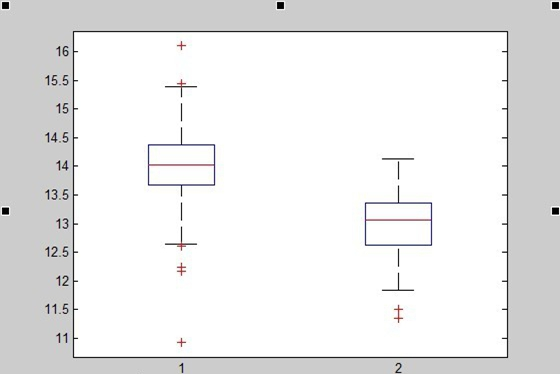1=function value of spacer; 2=function value of random spacer)

Now our model have worked as we wish to give a characteristic equation to the spacers!